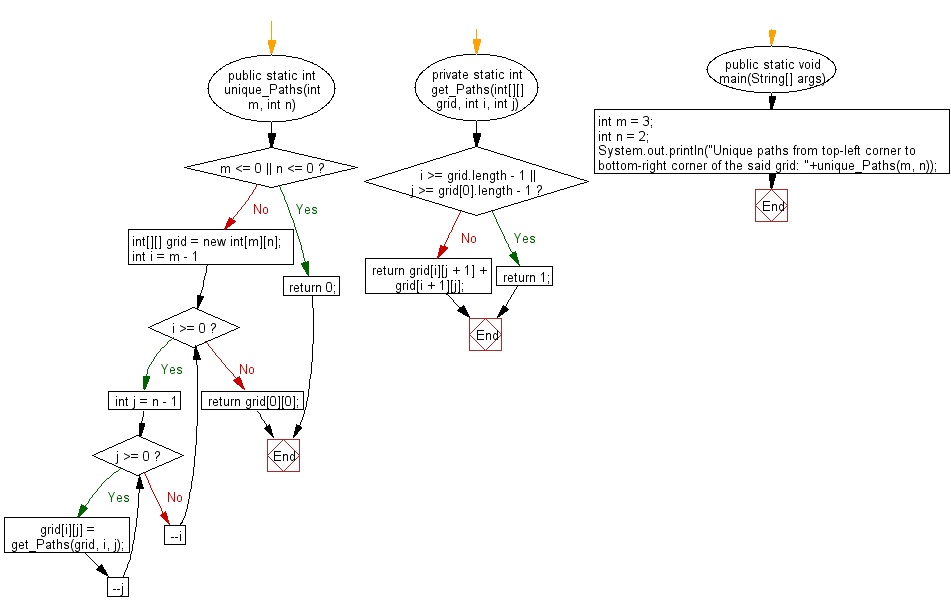﻿ Java: Paths from top-left corner to bottom-right of a grid# Java Exercises: Find possible unique paths from top-left corner to bottom-right corner of a specified grid

## Java Basic: Exercise-136 with Solution

Write a Java program to find possible unique paths from top-left corner to bottom-right corner of a given grid (m x n).

Note: You can move either down or right at any point in time.

Sample Solution:

Java Code:

``````public class Solution {
/**
* @param n, m: positive integer (1 <= n ,m <= 100)
* @return an integer
*/
public static int unique_Paths(int m, int n) {
if (m <= 0 || n <= 0) {
return 0;
}
int[][] grid = new int[m][n];
for (int i = m - 1; i >= 0; --i) {
for (int j = n - 1; j >= 0; --j) {
grid[i][j] = get_Paths(grid, i, j);
}
}
return grid;
}

private static int get_Paths(int[][] grid, int i, int j) {
if (i >= grid.length - 1 || j >= grid.length - 1) {
return 1;
}
return grid[i][j + 1] + grid[i + 1][j];
}

public static void main(String[] args) {
int m = 3;
int n = 2;
System.out.println("Unique paths from top-left corner to bottom-right corner of the said grid: "+unique_Paths(m, n));
}
}
```
```

Sample Output:

```Unique paths from top-left corner to bottom-right corner of the said grid: 3
```

Flowchart:Java Code Editor:

Company:

What is the difficulty level of this exercise?

Test your Programming skills with w3resource's quiz.

﻿

## Java: Tips of the Day

countOccurrences

Counts the occurrences of a value in an array.

Use Arrays.stream().filter().count() to count total number of values that equals the specified value.

```public static long countOccurrences(int[] numbers, int value) {
return Arrays.stream(numbers)
.filter(number -> number == value)
.count();
}
```

Ref: https://bit.ly/3kCAgLb